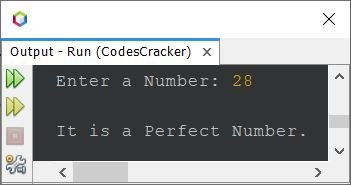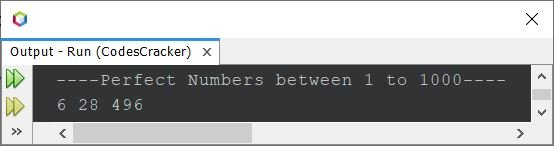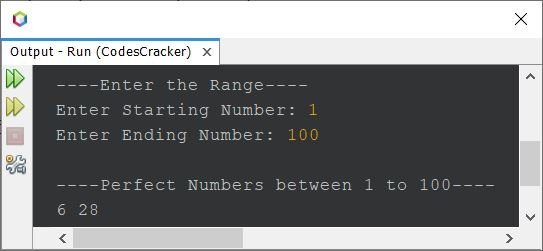# Perfect Number Program in Java

This article is created to cover perfect number program in Java. That is, the article contains some programs on perfect number. But before creating the perfect number program, let's first understand, what does it mean by perfect number.

A perfect number is a number that is equal to the sum of its positive divisors (excluding the number itself). For example, 6, 28, 496 etc. are perfect numbers. Because:

```6 = 1+2+3
= 6```

where 1, 2, and 3 are positive divisors of 6. Similarly:

```28 = 1+2+4+7+14
= 28```

## Check Perfect Number using for Loop

The question is, write a Java program to check whether a number entered by user at run-time, is a perfect number or not using for loop. The answer to this question, is the program given below:

```import java.util.Scanner;

public class CodesCracker
{
public static void main(String[] args)
{
int num, i, sum=0;
Scanner s = new Scanner(System.in);

System.out.print("Enter a Number: ");
num = s.nextInt();

for(i=1; i<num; i++)
{
if(num%i==0)
sum = sum+i;
}

if(sum==num)
System.out.println("\nIt is a Perfect Number.");
else
System.out.println("\nIt is not a Perfect Number.");
}
}```

The snapshot given below shows the sample run of above Java program, with user input 28 to check whether it is a perfect number or not:## Check Perfect Number using while Loop

Let's create the same program using while loop.

```import java.util.Scanner;

public class CodesCracker
{
public static void main(String[] args)
{
int num, i=1, sum=0;
Scanner s = new Scanner(System.in);

System.out.print("Enter a Number: ");
num = s.nextInt();

while(i<num)
{
if(num%i==0)
sum = sum+i;
i++;
}

if(sum==num)
System.out.println("\nIt is a Perfect Number.");
else
System.out.println("\nIt is not a Perfect Number.");
}
}```

The output produced by this program, is exactly same as of previous program.

Since in case of while loop, we need to do the initialization part before, and update part inside, the loop. Therefore, while declaring the variable i, I've initialized its value with 1, and inside the while loop, I've added one extra statement, that is i++; to increment the value of i by 1, at each execution of the loop.

## Find and Print Perfect Numbers between 1 to 1000

Here is another program used to find and print all perfect numbers between 1 and 1000. This program does not receive inputs from user.

```public class CodesCracker
{
public static void main(String[] args)
{
int num, start=1, end=1000, i, sum;

System.out.println("----Perfect Numbers between 1 to 1000----");
for(num=start; num<=end; num++)
{
sum = 0;
for(i=1; i<num; i++)
{
if(num%i==0)
sum = sum+i;
}
if(sum==num)
System.out.print(num+ " ");
}
}
}```

Here is its sample output:## Find Perfect Numbers in Given Range

Now this is the last program of this article, created using for loop to find and print all perfect numbers between a range provided by user at run-time of the program. This program is similar to previous program. The only difference is, the value of start and end variable is received by user.

```import java.util.Scanner;

public class CodesCracker
{
public static void main(String[] args)
{
long num, start, end, i, sum;
Scanner s = new Scanner(System.in);

System.out.print("----Enter the Range----");
System.out.print("\nEnter Starting Number: ");
start = s.nextLong();
System.out.print("Enter Ending Number: ");
end = s.nextLong();

if(start<end)
{
System.out.println("\n----Perfect Numbers between "+start+" to "+end+"----");
for(num=start; num<=end; num++)
{
sum = 0;
for(i=1; i<num; i++)
{
if(num%i==0)
sum = sum+i;
}
if(sum==num)
System.out.print(num+ " ");
}
}
else
System.out.println("\nInvalid Input!");
}
}```

Here is its sample run with user input 1 as starting, and 100 as ending number to print all perfect numbers between 1 to 100:Java Online Test

« Previous Program Next Program »